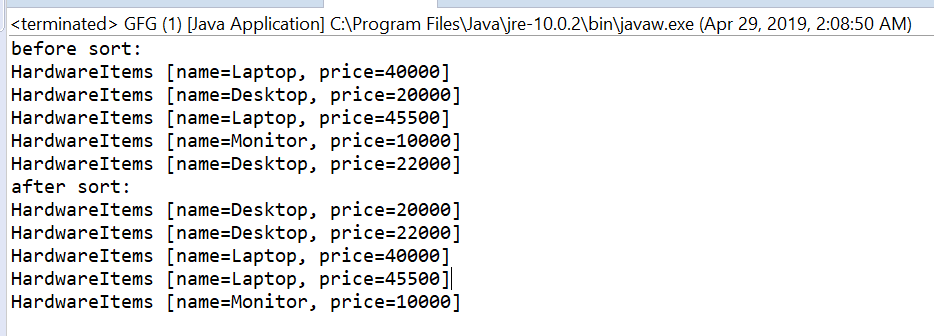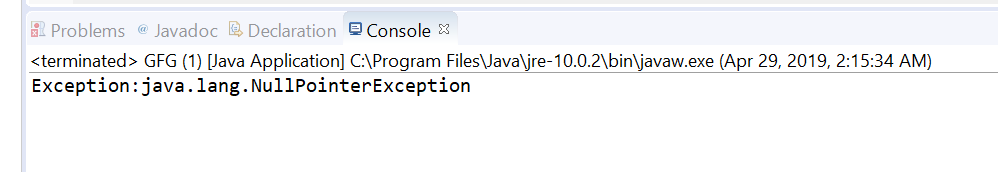# Comparator thenComparingInt() method in Java with examples

The thenComparingInt(java.util.function.ToIntFunction) method of Comparator Interface in Java returns a lexicographic-order comparator with a function that extracts a int sort key.

Syntax:

```default Comparator <T>
thenComparingInt(ToIntFunction <T> keyExtractor)
```

Parameters: This method accepts keyExtractor which is the function used to extract the Int sort key.

Return value: This method returns a lexicographic-order comparator composed of this and then the Int sort key.

Exception: This method throws NullPointerException if the argument is null.

Below programs illustrate thenComparingInt(java.util.function.ToIntFunction) method:
Program 1:

 `// Java program to demonstrate Comparator ` `// thenComparingInt(ToIntFunction) method ` ` `  `import` `java.util.Arrays; ` `import` `java.util.Collections; ` `import` `java.util.Comparator; ` `import` `java.util.List; ` ` `  `public` `class` `GFG { ` `    ``public` `static` `void` `main(String... args) ` `    ``{ ` ` `  `        ``List list = getItems(); ` `        ``System.out.println(``"before sort:"``); ` `        ``list.forEach(System.out::println); ` ` `  `        ``// Apply sorting and ` `        ``// also apply thenComparingInt() ` `        ``Collections ` `            ``.sort( ` `                ``list, ` `                ``Comparator ` `                    ``.comparing(HardwareItems::getName) ` `                    ``.thenComparingInt(HardwareItems::getPrice)); ` ` `  `        ``System.out.println(``"after sort:"``); ` `        ``list.forEach(System.out::println); ` `    ``} ` ` `  `    ``private` `static` `List getItems() ` `    ``{ ` `        ``return` `Arrays.asList( ` `            ``new` `HardwareItems(``"Laptop"``, ``40000``), ` `            ``new` `HardwareItems(``"Desktop"``, ``20000``), ` `            ``new` `HardwareItems(``"Laptop"``, ``45500``), ` `            ``new` `HardwareItems(``"Monitor"``, ``10000``), ` `            ``new` `HardwareItems(``"Desktop"``, ``22000``)); ` `    ``} ` ` `  `    ``private` `static` `class` `HardwareItems { ` `        ``private` `String name; ` `        ``private` `int` `price; ` ` `  `        ``public` `HardwareItems(String name, ``int` `price) ` `        ``{ ` `            ``this``.name = name; ` `            ``this``.price = price; ` `        ``} ` ` `  `        ``public` `String getName() ` `        ``{ ` `            ``return` `name; ` `        ``} ` ` `  `        ``public` `int` `getPrice() ` `        ``{ ` `            ``return` `price; ` `        ``} ` ` `  `        ``@Override` `        ``public` `String toString() ` `        ``{ ` `            ``return` `"HardwareItems [name="` `                ``+ name ` `                ``+ ``", price="` `                ``+ price + ``"]"``; ` `        ``} ` `    ``} ` `} `

The output printed on console of IDE is shown below.
Output:You can see in example first sorting is done on name wise and if the name is same then price wise which is int type.

Program 2:

 `// Java program to demonstrate Comparator ` `// thenComparingInt(ToIntFunction)  method ` ` `  `import` `java.util.Arrays; ` `import` `java.util.Comparator; ` `import` `java.util.List; ` ` `  `public` `class` `GFG { ` ` `  `    ``public` `static` `void` `main(String... args) ` `    ``{ ` ` `  `        ``List list ` `            ``= Arrays.asList(``1.12``, ``2.4``, ``3.43``, ` `                            ``4.343``, ``5.434``); ` ` `  `        ``try` `{ ` ` `  `            ``// apply thenComparingInt ` `            ``Comparator.comparing(list::get) ` `                ``.thenComparingInt(``null``); ` `        ``} ` `        ``catch` `(Exception e) { ` ` `  `            ``System.out.printf(``"Exception:"` `+ e); ` `        ``} ` `    ``} ` `} `

The output printed on console is shown below.
Output:Attention reader! Don’t stop learning now. Get hold of all the important DSA concepts with the DSA Self Paced Course at a student-friendly price and become industry ready.

My Personal Notes arrow_drop_upCheck out this Author's contributed articles.

If you like GeeksforGeeks and would like to contribute, you can also write an article using contribute.geeksforgeeks.org or mail your article to contribute@geeksforgeeks.org. See your article appearing on the GeeksforGeeks main page and help other Geeks.

Please Improve this article if you find anything incorrect by clicking on the "Improve Article" button below.

Article Tags :
Practice Tags :

Be the First to upvote.

Please write to us at contribute@geeksforgeeks.org to report any issue with the above content.